Chengling Gou (2006)

The Simulation of Financial Markets by an Agent-Based Mix-Game Model

Journal of Artificial Societies and Social Simulation vol. 9, no. 3
< https://www.jasss.org/9/3/6.html >

Received: 06-Dec-2005    Accepted: 13-May-2006    Published: 30-Jun-2006###Abstract

This paper studies the simulation of financial markets using an agent-based mix-game model which is a variant of the minority game (MG). It specifies the spectra of parameters of mix-game models that fit financial markets by investigating the dynamic behaviors of mix-game models under a wide range of parameters. The main findings are (a) in order to approach efficiency, agents in a real financial market must be heterogeneous, boundedly rational and subject to asymmetric information; (b) an active financial market must be dominated by agents who play a minority game; otherwise, the market would die; (c) the system could be stable if agents who play a majority game have a faster learning rate than those who play a minority game; otherwise, the system could be unstable. The paper then induces the rules for simulating financial markets with mix-game models and gives an example. Finally, the appendix of this paper presents background information about 'El Farol bar', MG and mix-games.

Keywords:
Financial Markets, Simulation, Minority Game, Mix-Game

###Introduction

1.1
In the last few decades, economic finance has seen a paradigm shift from modeling with homogeneous agents to heterogeneous agents. In the traditional approach, mathematics has been the main tool of analysis, but it can only deal with simple analytically tractable models with homogeneous, perfectly rational agents. For the new paradigm, computational and numerical methods have become an important tool of analysis, with agent-based simulation models supported by more and more powerful computational technology (Judd and Tesfatsion 2005; Johnson et al 2003; Luna and Perrone 2002). Very recently LeBaron reviewed agent-based financial market models (Judd and Tesfatsion 2005). In addition to economists, there are some econophysicists who have contributed to agent-based financial market models. Econophysicists investigate financial markets from the point of view of statistical physics which focuses on the underlying mechanisms of financial markets other than the details of financial markets. Minority game (MG) extended from Arthur's 'El Farol bar' problem by Challet and Zhang (1997) is an example among such effort. The further details about 'El Farol bar' problem and minority game are available in the appendix.

1.2
MG model attracts a lot of following studies (Coolen 2005). Given MG's richness and yet underlying simplicity, MG has also received much attention as a financial market model (Johnson et al 2003; Jefferies and Johnson 2001). Johnson and coworkers extended MG by allowing a variable number of active traders at each time-step — they called their modified game as a Grand Canonical Minority Game (GCMG). GCMG, and to a lesser extent the standard MG itself, can reproduce the stylized facts of financial markets, such as volatility clustering and fat-tail distributions. However, only with certain spectrum of parameters of standard MG and its variants, can they simulate financial markets. But there are few papers that explicitly address this issue (Gou 2006; Gou 2005). This paper investigates this issue by studying the simulation of financial markets with agent-based mix-game models in which there are two groups of agents: Group 1 plays a majority game which is the opposite of a minority game; while Group 2 plays a minority game. It specifies the spectra of parameters of mix-game models that fit financial markets by investigating the dynamic behaviors of mix-game models under a wide range of parameters. The main findings are (a) in order to approach efficiency, agents in a real financial market must be heterogeneous, boundedly rational and subject to asymmetric information; (b) an active financial market must be dominated by agents who play a minority game, otherwise, the market would die; (c) the system could be stable if agents who play a majority game have a faster learning rate than those who play a minority game; otherwise, the system could be unstable.

1.3
This paper is organized as following: Section 2 introduces mix-game models and simulation conditions. Section 3 reports the simulation results and discussions. Section 4 induces the rules for simulating financial markets with mix-game models and gives an example. Section 5 presents the conclusion of this study. The detail description of the 'El Farol bar' problem, Challet and Zhang's MG model and the motivation of mix-games is given in the appendix.

###Mix-game models and simulation conditions

2.1
Inspired by MG and its two variants (Johnson et al 1999; Marsili 2001), the author developed a mix-game model in order to find a better model to simulate financial markets. The code is here. A mix-game model is a modification of MG so that they have the similar structures. In a mix-game, there are two groups of agents: Group 1 plays a majority game, and Group 2 plays a minority game. N is the total number of agents and N1 is the number of agents in Group 1. The system resource is R=0.5×N. All agents compete in the system for the limited resource R. T1 and T2 are the time horizons of the two groups, during which agents count their own performances so that these parameters represent the learning rates of agents who learn from their own past performance. And m1 and m2 denote the historical memories of the two groups, respectively. The difference between a mix-game and mixed Minority/Majority Games (Marsili 2001) is that the two groups in a mix-game have different bounded abilities to deal with historical information and to count their own performances. Therefore, agents are more heterogeneous in a mix-game than in MG and its two variants mentioned above. This feature of a mix-game represents that agents have bounded rationality (Shleifer 2000).

2.2
Only global information available to agents is a common bit-string "memory" of m1 or m2 most recent competition outcomes. A strategy consists of a response, i.e. 0 or 1, to each possible bit string; hence there are 22m1 or 22m2 possible strategies for Group 1 or Group 2, respectively, which form full strategy spaces (FSS). At the beginning of a game, each agent is assigned s strategies and keeps them unchanged during the game. After each turn, agents assign one point (virtual) to a strategy that would have predicted the correct outcome. For agents in Group 1, they will reward their strategies one point if they are in the majority side; on the other hand, for agents in Group 2, they will reward their strategies one point if they are in the minority side. Agents collect the virtual points for their strategies over the time horizon T1 or T2, and agents use their strategy that has the highest virtual point at each turn. If there are two strategies that have the highest virtual point, agents use coin toss to decide which strategy to be used. Excess demand D[(t)-] is equal to the number of ones (buying) minus the number of zeros (selling).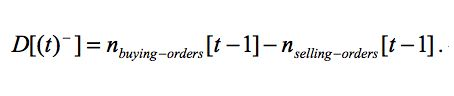(1)

2.3
According to a widely accepted assumption that excess demand exerts a force on the price of an asset and the changes of prices are proportional to the excess demands in a financial market (Bouchaud and Cont 1998; Farmer 2002), the time series of prices of an asset P[(t)] can be calculated based on the time series of excess demands.(2)

2.4
Volatility of the time series of prices is represented by the variance of the increases of prices. Local volatility (Vol) is calculated at every time-step by calculating the variance of the increases of prices within a small time-window d. If chosen λ = 1 for simplicity, the increase of prices is just the excess demand. Therefore, we obtain the following formula: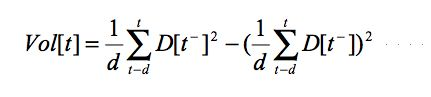(3)

2.5
In simulations, the distribution of initial strategies of agents is randomly uniform in FSS and remains unchanged during a game. Each agent has two strategies, i.e. s=2. The simulation running time-steps are 3000. The window length of local volatility is d=5.

###Simulation results and discussions

3.1
In order to specify the spectra of parameters of mix-games that fit financial markets, the following subsections investigate three groups of parameters: (a) historical memories (m1, m2); (b) fraction of Group 1 (N1/N); (c) time horizons (T1, T2).

#### Dynamics of mix-games with different configurations of historical memories

3.2
In order to study the differences of dynamic behaviors of mix-game models with different configurations of historical memories as shown in Figure 1, simulations have been done under the condition of T1=T2=12, N=201, N1=72 to investigate the correlations between volatilities of price time series and average winnings of each group.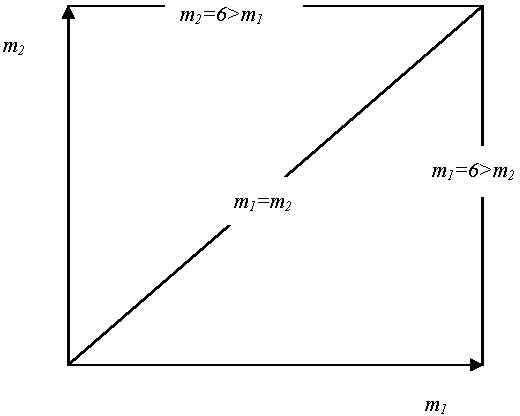Figure 1. Three configurations of historical memories

3.3
It is found that the correlations between average winnings of each group and means of local volatilities are different when historical memories change under three configurations: m1=m2, m1 < m2=6 and m2 < m1=6.
 Table 1: Correlations among R1, R2 and Vol1 when m2=6, and m1 increases from 1 to 6 R 1 R 2 Vol1 R 1 1 R 2 0.982 1 Vol1 0.985 0.996 1

3.4
If m1 < m2=6, both the means of local volatilities and the average winnings of these two groups increase when m1 decrease from 6 to 1. This implies that agents with smaller memories in Group 1 can not only improve their own performance but also can benefit to agents in Group 2. The cooperation between Group 1 and Group 2 emerges in this case. Table 1 shows that correlations among R1, R2 and Vol1 are greatly positive, where R1 and R2 represent the average winnings of Group 1 and Group 2, respectively, and Vol1 represents the means of local volatilities. These results mean that the improvement of agent performances accompanies with the decrease of system efficiency in this case. Agents can benefit from greater system fluctuations. This is accordant with the reality of financial markets. Therefore, mix-game models with this configuration of historical memories can be considered as candidates for modeling financial markets.
 Table 2: Correlations among R1, R2 and Vol2 when m1=6, and m2 increases from 1 to 6 R1 R2 Vol2 R1 1 R2 -0.246 1 Vol2 0.148 -0.892 1

3.5
If m1=6 > m2, the average winnings of Group 2 decrease, but the average winnings of Group 1 do not change greatly, while the means of local volatilities increase when m2 decreases from 6 to 1. Table 2 shows that the average winnings of Group 2 (R2) strongly negatively correlate with the system volatilities (Vol2), but the average winnings of Group 1 (R1) just slightly positively correlate with the system volatilities (Vol2). This result is due to the uncorrelated strategy spaces between these two groups (Gou 2006). That is to say, these two groups behave independently.
 Table 3: Correlations among R1, R2 and Vol3 when m1=m2, and they increase from 1 to 6 R1 R2 Vol3 R1 1 R2 0.977 1 Vol3 -0.859 -0.737 1

3.6
If m1=m2, the average winnings of these two groups decrease, but the means of local volatilities increase when m1 and m2 decreases from 6 to 1. Table 3 shows that the average winnings of Group 1 (R1) and Group 2 (R2) negatively correlate with the system volatilities (Vol3). In this case, average winnings of these two groups are similar to that of MG, but the volatility decreases greatly just due to their anti-correlated strategy spaces (Gou 2006).

3.7
The above results suggest that mix-games with m1 being smaller than m2=6 can be used to simulate financial markets. That is to say, for simulating a financial market with a mix-game, agents in Group 1 must have less historical information than in Group 2. This implies that a real financial market consists of heterogeneous agents with bounded rationality and subject to asymmetric information.

#### Dynamics of mix-games with different fractions of Group 1

3.8
To study the influence of the fraction of agents in Group 1 (N1/N) on dynamic behaviors of mix-game models, simulations have been done under the condition of m1 < m2=6. Through simulations, it is found that local volatilities drop to zeros in most cases when N1/N is greater than 0.5 with some initial strategy distributions. This phenomenon is also influenced by T1.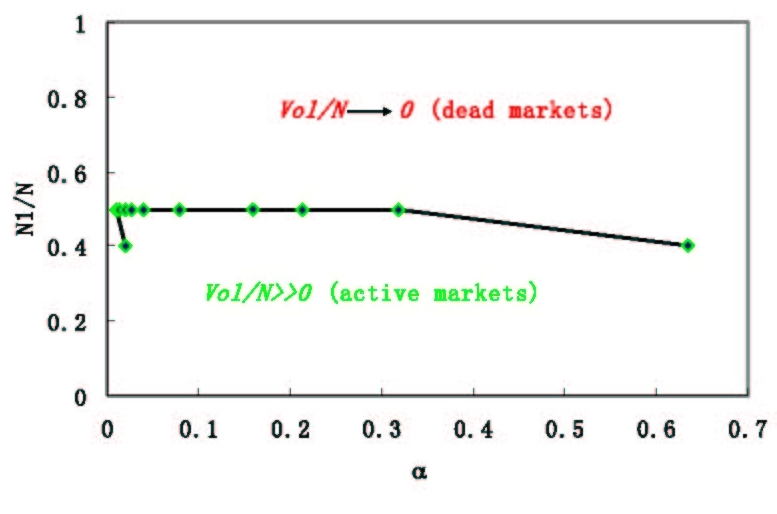Figure 2. Two-phase phenomenon of local volatilities under simulation condition of m1 < m2, where α = 2m1 / N

3.9
Figure 2 shows the two-phase phenomenon of local volatilities under the condition of m1 < m2, where α=2 m1 / N . The fact of local volatilities dropping to zeros means that a market is 'dead'. The reason for this phenomenon is that all agents quickly adapt themselves to using one strategy and stick to it, i.e. all agents are 'frozen' in this case. This phenomenon can be found frequently in pure majority games, and the smaller the historical memory m is, the easier agents become 'frozen'. Therefore, we can say that the characteristic of a minority game dominates in the regime of N1/N < 0.5, but the characteristic of a majority game dominates in the regime of Ni / N ≥ 0.5 except two point as shown in Figure 2 where the phases change at N1/N=0.4.

3.10
This result suggests that N1/N be smaller than 0.5 if one use a mix-game to simulate a financial market. That is to say, an active financial market must be dominated by agents in Group 2 who play a minority game; otherwise, the market would die.

#### Dynamics of mix-games with different time horizons

3.11
T1 or T2 is used to record the virtual point of each strategy of agents, i.e. T1 or T2 records the private information about agent strategies, according to which, agents make their own decisions. The greater T1 or T2, the more past information deposits and influences decision-making of agents. Therefore, these parameters represent the learning rates of agents who learn from their own past performance. The greater T1 or T2 is, the slower the learning rate is. A fast learning rate means that agents can adapt themselves to their environment fast.

3.12
To investigate the influence of time horizons (T1, T2) on dynamic behaviors of mix-game models, simulations have been done under the condition of m1=3, m2=6, N=201, N1=72 and s=2. One example of simulations is shown in Figure 3 that plots the relations between means of local volatilities and time horizon T1 (T2) when T2=36 (T1=36), m1=3, m2=6, N=201, N1=72 and s=2. It is found that means of local volatilities are stable if T1 ≤ T2. Otherwise, the means of local volatilities are unstable and greater than the stable values. That is to say, there are two areas in T1-T2 space as shown in Figure 4: one is stable area where T1 ≤ T2; the other is unstable area where T1>T2. This result implies that the system could be stable if agents in Group 1 who play a majority game have a faster learning rate than those in Group 2 who play a minority game; otherwise, the system could be unstable. This finding can be used to explain the phenomenon of local volatility clustering: the system will be more fluctuating if agents in Group 2 adapt themselves to a faster learning rate or if agents in Group 1 adapt themselves to a slower learning rate; in contrast, the system will be more stable if agents in Group 2 switch back to a slower learning rate or if agents in Group 1 switch back to a faster learning rate.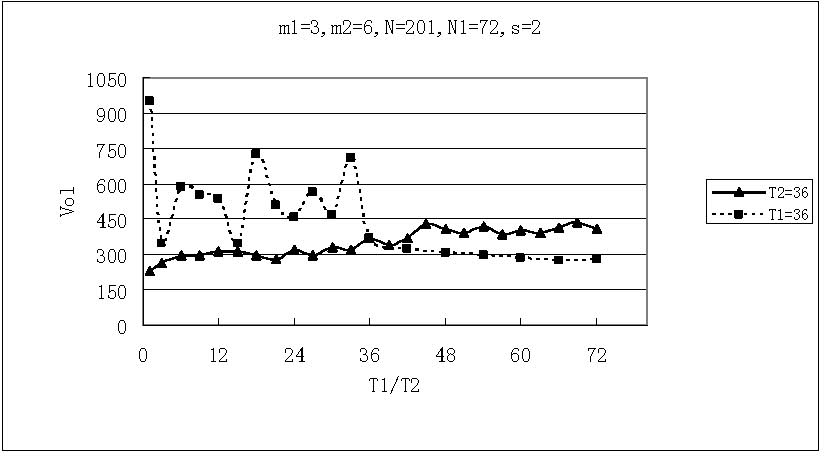Figure 3. Relations between means of local volatilities and time horizon T1 (T2) when T2=36 (T1=36), m1=3, m2=6, N=201, N1=72 and s=2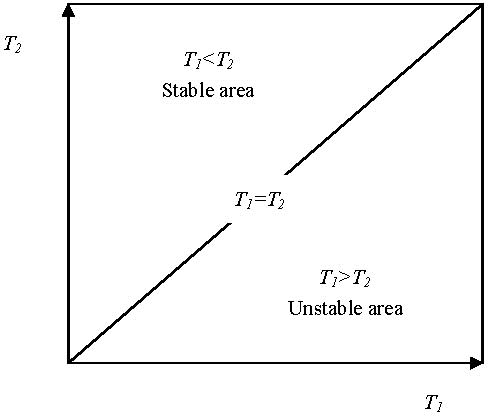Figure 4. Two-phase phenomenon of local volatilities in T1-T2 space under simulation condition of m1=3, m2=6, N=201, N1=72 and s=2

###Modeling rules and an example

#### Modeling rules

4.1
From the previous sections, one can find out that mix-games with some parameters can reproduce the stylized features of financial markets, but some fail to do so. Therefore, one needs to choose parameters of m1, m2, T1, T2, N and N1 if he or she uses mix-games to model financial markets. The following aspects need to be considered:
• First make sure the time series of a mix-game can reproduce the stylized facts of financial markets by choosing proper parameters: (a) m1 < m2=6; (b) T1 ≤ T2; (c) N1/N<0.5;
• Second pay attention to the fluctuation of local volatilities, and ensure that the median of local volatilities of a mix-game is similar to that of the target time series;
• Third make sure the log-log plot of absolute returns look similar.

#### Simulating Shanghai Index daily with mix-game models

4.2
Since the median value of local volatilities of Shanghai Index daily data from 1992/01/02 to 2004/03/19 is 222, two parameter configurations of mix-games have the similar median values of local volatilities; one configuration is m1=3, m2=6, T1=12, T2=60, N=201, N1=40 and s=2; the other is m1=4, m2=6, T1=T2=12, N=201, N1=72 and s=2.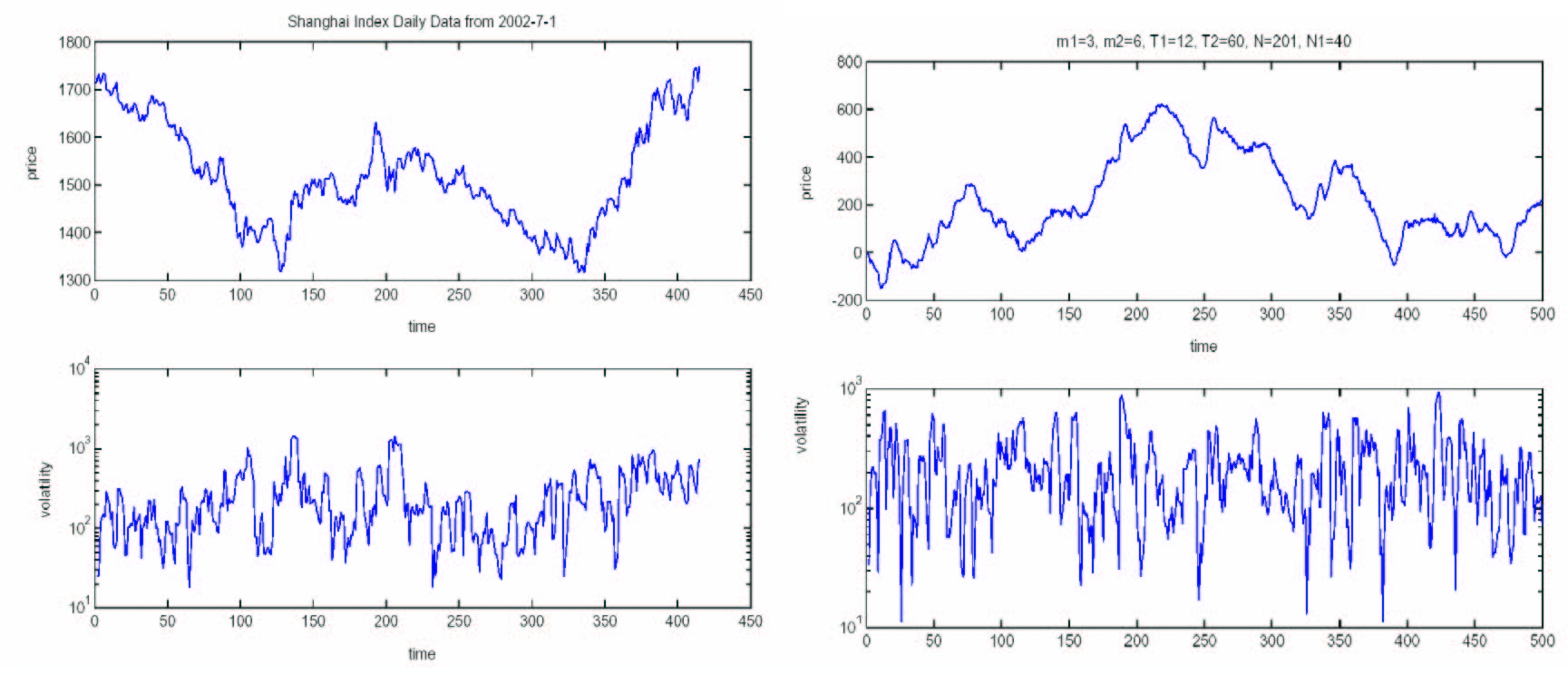Figure 5. Time series and local volatilities of Shanghai Index daily data and the mix-game with parameters of m1=3, m2=6, T1=12, T2=60, N=201, N1=40 and s=2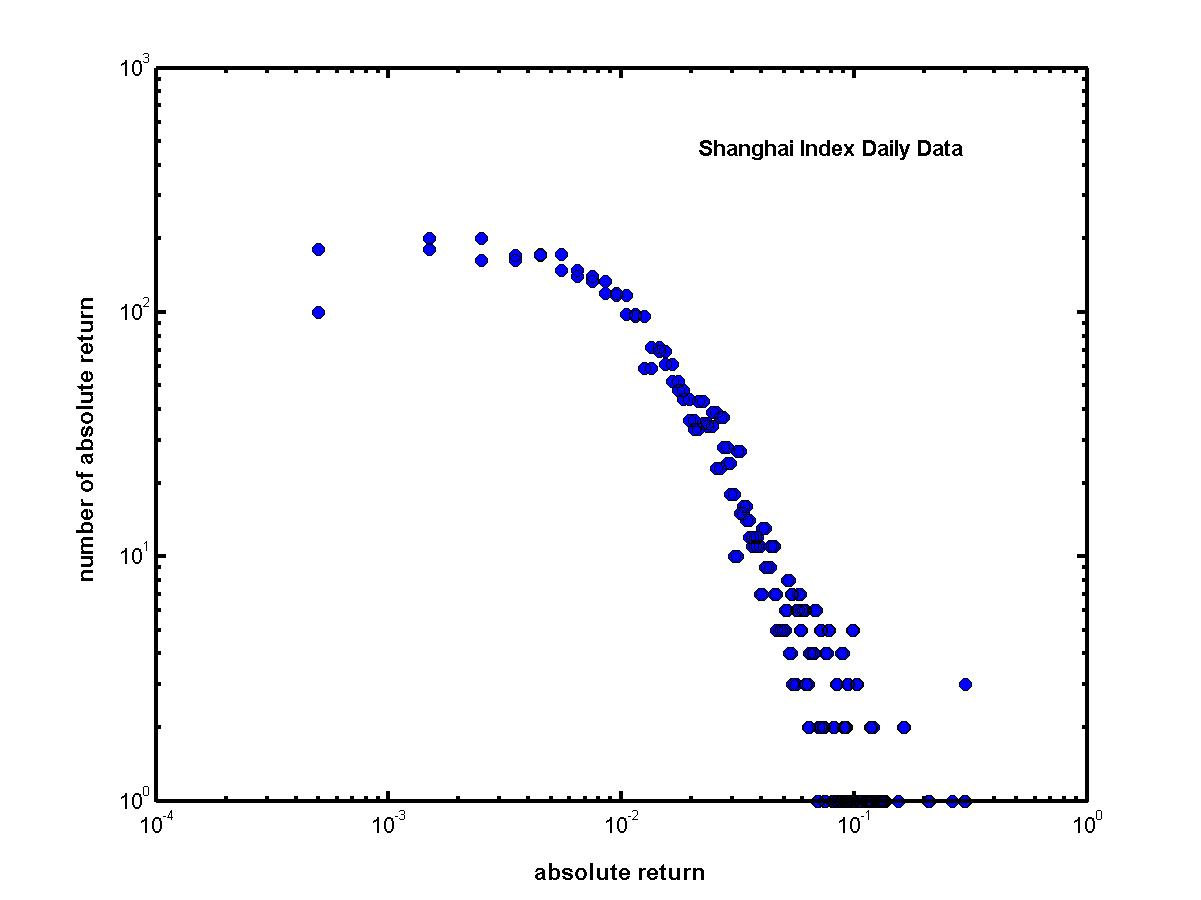Figure 6. Log-log plot of Shanghai Index daily absolute returns that is non-Gaussian (Yang 2004)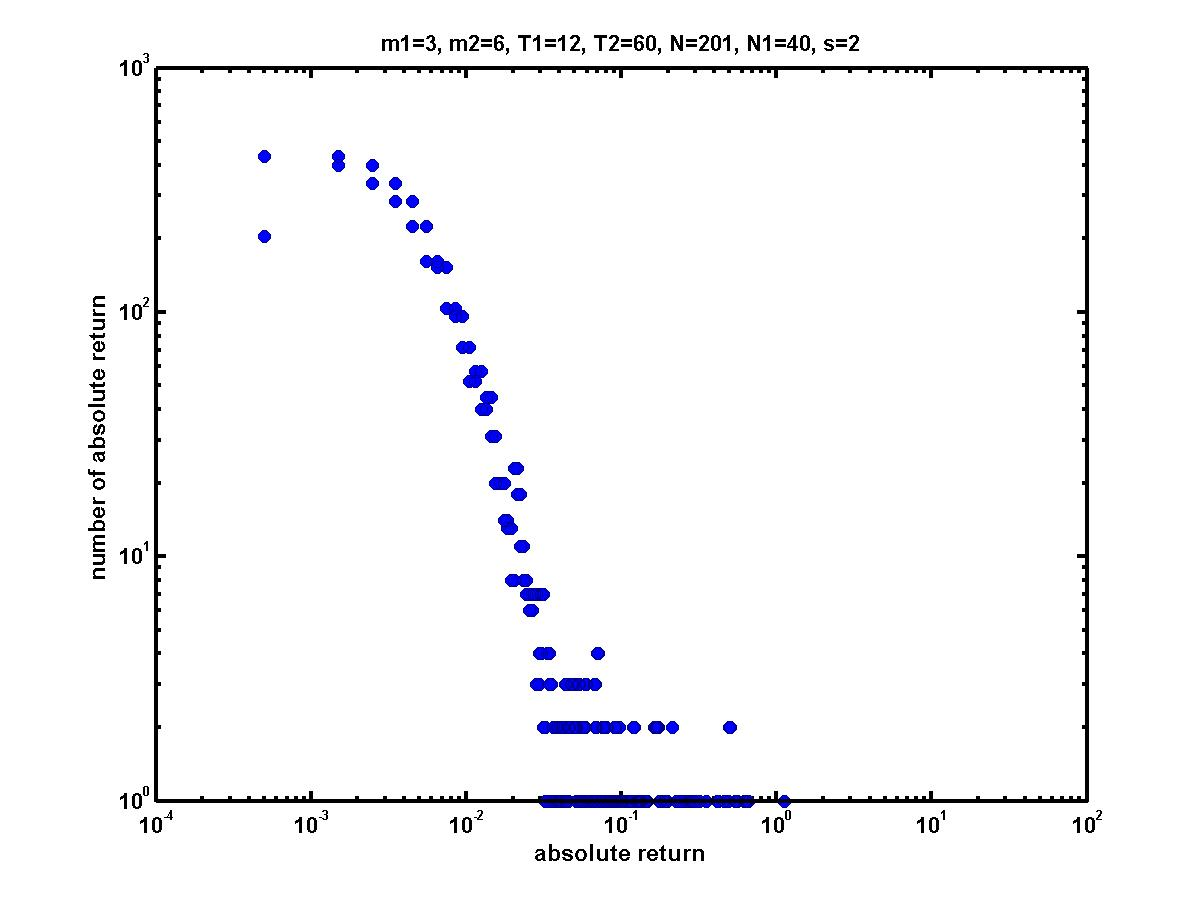Figure 7. Log-log plot of the mix-game absolute returns with parameters of m1=3, m2=6, T1=12, T2=60, N=201, N1=40 and s=2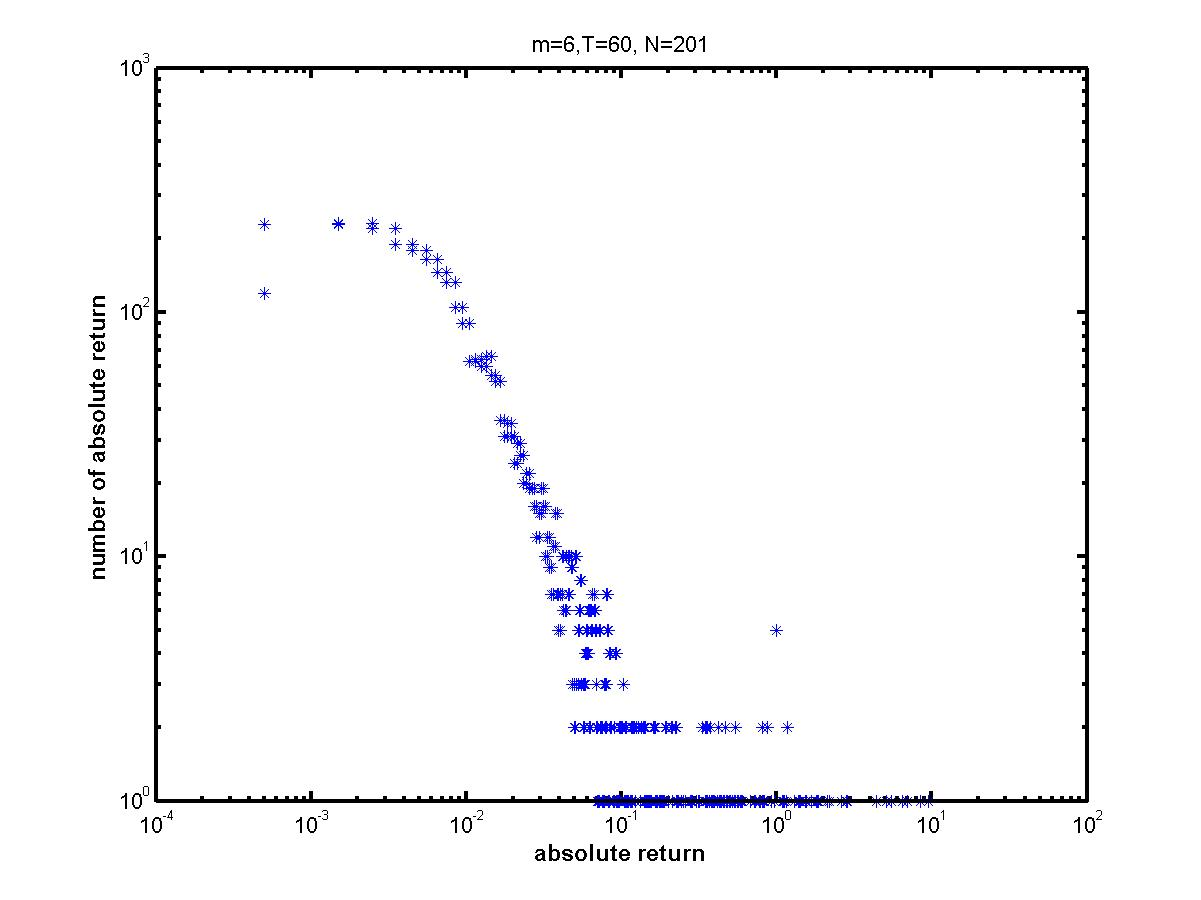Figure 8. Log-log plot of the MG absolute returns with parameters of m=6, T=60 and N=201 and s=2

4.3
Figure 5, Figure 6 and Figure 7 show that the mix-game with m1=3, T1=12, m2=6, T2=60, N1=40, N=201 and s=2 can reproduce the similar stylized facts of Shanghai stock market. So does the mix-game with m1=4, m2=6, T1=T2=12, N=201, N1=72 and s=2. As shown in Figure 8, the MG with m=6, T=60, N=201 and s=2 has a much fatter tail in log-log plot of its absolute returns than that of Shanghai index daily-data. Therefore, the mix-games behave better than the MG. for simulating Shanghai Index.

4.4
It is well known that returns are uncorrelated in real financial markets, and this argument is also held for Shanghai Index despite Shanghai stock market is an emerging market (Johnson et al 2003). Does the mix-game with m1=3, T1=12, m2=6, T2=60, N1=40, N=201 and s=2 displays this feature? Figure 9 presents the simulation result about the autocorrelations of logarithmic returns of the mix-game with m1=3, T1=12, m2=6, T2=60, N1=40, N=201 and s=2, and shows that the return process only displays weak autocorrelation for the first few lags and quickly drops to zeros for longer lags.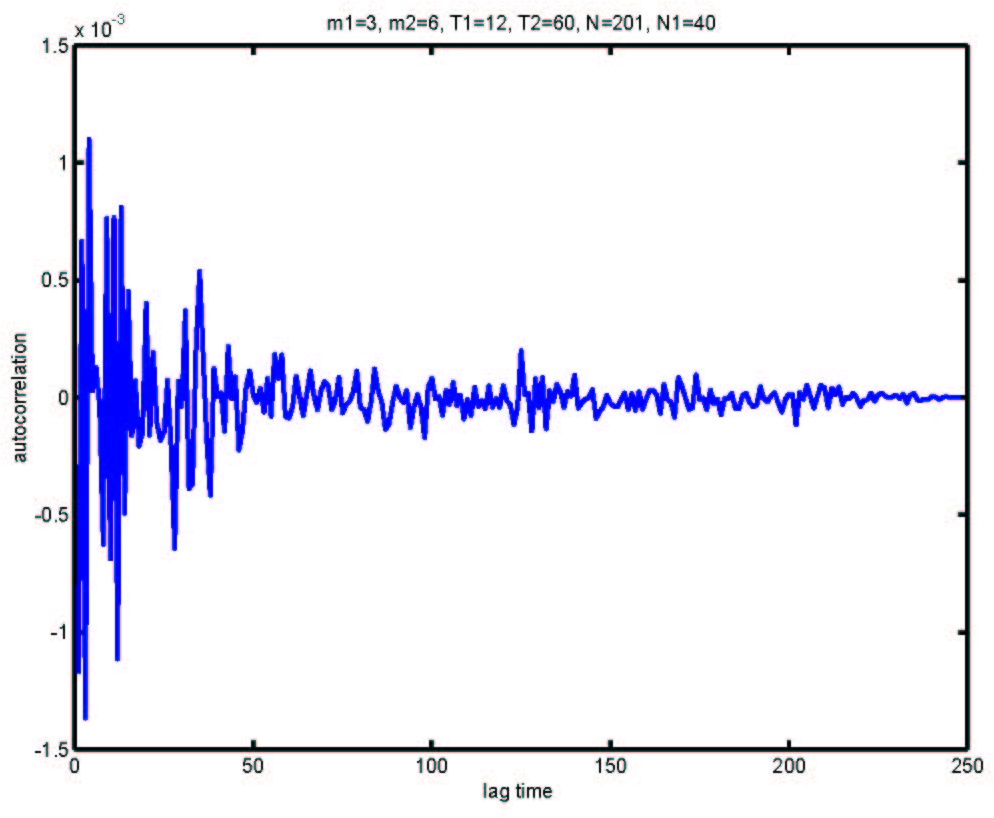Figure 9. Autocorrelations of logarithmic returns of the mix-game with m1=3, T1=12, m2=6, T2=60, N1=40, N=201 and s=2

###Conclusion

5.1
This study reveals that mix-games can be used to model financial markets subject to the following parameter configurations: (a) m1 < m2=6; (b) T1 ≤ T2; (c) N1/N < 0.5, within which the mix-games can reproduce the stylized facts of real financial markets. This result suggests that a real financial market consists of heterogeneous agents with bounded rationality and subject to asymmetric information. For simulation of Shanghai Index daily data from 1992/01/02 to 2004/03/19 with mix-games, there are two applicable parameter configurations: one is m1=3, m2=6, T1=12, T2=60, N=201, N1=40 and s=2, the other is m1=4, m2=6, T1=T2=12, N=201, N1=72 and s=2. Therefore, we can conclude that a mix-game can be a potentially good model to simulate a real financial market.

###Appendix

A.1
'El Farol bar' problem was proposed by Arthur (1999) in 1994. The El Farol bar is in Santa Fe. Every evening, there are 100 customers hoping to go to the bar, but the environment of the bar will be uncomfortable if the number of customers exceeds 60. In this case, customers prefer to stay at home so that every customer has to predict if there are more than 60 people in the bar. The difficulty for their predictions is that every customer does not know any other's decision but only know the historical information about the number of attending people every evening and the correct rate of his/her own decisions. Therefore, the customers face the problem of decision-making under incomplete information. In such case, customers have to use inductive reasoning to make decisions and learn from their own past performance.

A.2
Another interesting characteristic of 'El Farol bar' problem is that there is the internal frustration in 'El Farol bar' problem. It is impossible to find a model that can give a 100 percent accurate rate of predictions. If there was such a model, everyone would use it and make the same decision so that all customers would go to the bar or stay at home at the same time. In this way, this model fails to give correct predictions. Therefore, customers have to use different models to make their own decisions. The characteristic of frustration forces customers to have diversified anticipations. In financial markets, investors face the same problem as the customers in 'El Farol bar' problem. Hence, investors also have to use inductive reasoning and have diversified anticipations.

A.3
Inspired by 'El Farol bar' problem, Challet and Zhang (1997) proposed minority game (MG). MG comprises an odd number of agents (investors) choosing repeatedly between the options of buying (1) and selling (0) a quantity of a risky asset. Agents continually try to make the minority decision, i.e. buying assets when the majority of other agents are selling and selling when the majority of other agents are buying. In this way, they can benefit from being in the minority side. During the process of the game, agents have two kinds of information: one is public information that records the most recent competition outcomes with historical memory m; the other is private information that agents have by counting their own performance during a time horizon T. Finite T and m mean that agents only have bounded abilities to remember and analyze information.

A.4
We can choose '1' and '0' to record the past history of competition by assigning a '0' to a outcome in which the majority of agents choose their actions of selling (0) and assigning a '1' to a outcome in which the majority of agents choose their actions of buying (1). So there are 2m pieces of public historical information for historical memory m in total. For example, '101' is a piece of public historical information for m=3. Agents use strategies to reflect history information to their actions (predictions). Table 4 gives a sample of agent strategies for m=2. There are 22mstrategies in total for historical memory m, which form full strategy space (FSS). But every agent only has a few strategies that are assigned to him/her at the beginning of a game and unchanged during the game. After each competition round, every agent evaluates his/her strategies by assigning one virtual point to a strategy which would have predicted the correct outcome, i.e. the agent would have been in the minority side if he/shi had taken an action according to this strategy. Otherwise, he/she assigns zero to his/her strategies. Agents collect virtual points for their strategies during time-horizon T. At the beginning of each round, agents choose the strategy that has the highest virtual point. If there are two strategies that have the same highest virtual points, agents use coin-toss to decide which strategy to be used.
 Table 4: A sample of agent's strategies (m=2) Historical information prediction 00 1 01 0 10 0 11 1

A.5
MG attracted many following study (Johnson et al 2003; Coolen 2005). However, there are some weaknesses in MG. First, the diversity of agents is limited, since agents all have the same historical memory and time-horizon. Second, in real markets, some agents are "fundamentalist", who effectively play a minority game; while the others are trend-followers, i.e. "noise traders", who effectively play a majority game that is the opposite of a minority game (Lux 1995; Lux and Marchesi 1999; Shleifer 2000). In order to create an agent-based model that more closely mimics a real financial market, the author modified MG by dividing agents into two groups: each group has different historical memories and time-horizons; one group plays a minority game and the other plays a majority game. For this reason, we will refer to this system as a 'mix-game' model. The highlight of a mix-game model is that the two groups of agents have different bounded abilities to deal with historical information and to count their own performance. This feature of mix-games represents that agents have different bounded rationality.

###References

ARTHUR W.B. (1999) Science 284, 107.

BOUCHAUD J.P. and Cont, R. (1998) Eur. Phys. J. B 6 543.

CHALLET, D. and Zhang, Y. C. (1997) Phyisca A 246, 407.

COOLEN A C C (2005) The Mathematical Theory of Minority Games, Oxford University Press, Oxford.

FARMER J.D. (2002) Industrial and Corporate Change vol. 11, 895-953.

GOU C. (2005) arXiv:physics/0508056.

GOU C. (2006) Chinese Physics 15 1239.

JEFFERIES P. and Johnson N. F. (2001) Oxford Center for Computational Finance working paper: OCCF/010702.

JOHNSON N. F., Hui P. M., Zheng D. and Hart M. (1999) J. Phys. A: Math. Gen. 32 L427-L431.

JOHNSON Neil F.,Jefferies P., and Hui P. M. (2003) Financial Market Complexity, Oxford University Press, Oxford.

JUDD K.L. and Tesfatsion L. edited (2005) Handbook of Computational Economics, Volume 2: Agent-Based Computational Economics, Elsevier Science B.V.

LUNA F. and Perrone A. edited (2002) Agent-based Methods in Economics and Finance: Sinulations in Swarm, Kluwer Academic Publisher, Norwell, Massachusetts.

LUX T. (1995) Economic Journal 105 881

LUX T. and Marchesi M. (1999) Nature 397 498

MARSILI M. (2001) Physica A 299 93.

SHLEIFER A. (2000) Inefficient Markets: an Introduction to Behavioral Financial, Oxford University Press, Oxford, UK.

YANG C. (2004) thesis of Beijing University of Aeronautics and Astronautics.